Physics
Fox

### Summary

• Potential difference (also known as voltage) is defined as follows: Potential difference is the difference in potential energy for each electron.

• Potential difference has the symbol V, and the unit of Volts: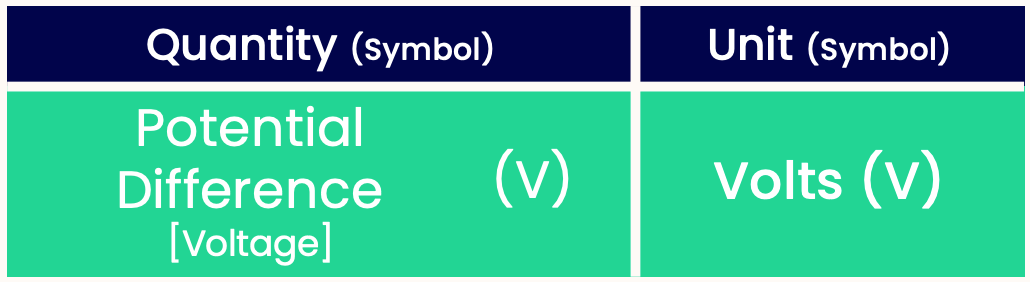• Higher-voltage batteries pump electrons to a higher electrical energy than lower-voltage batteries (e.g. 12V car battery vs 1.5V AA battery). Therefore, higher-voltage batteries can be used to power more energy-intensive devices.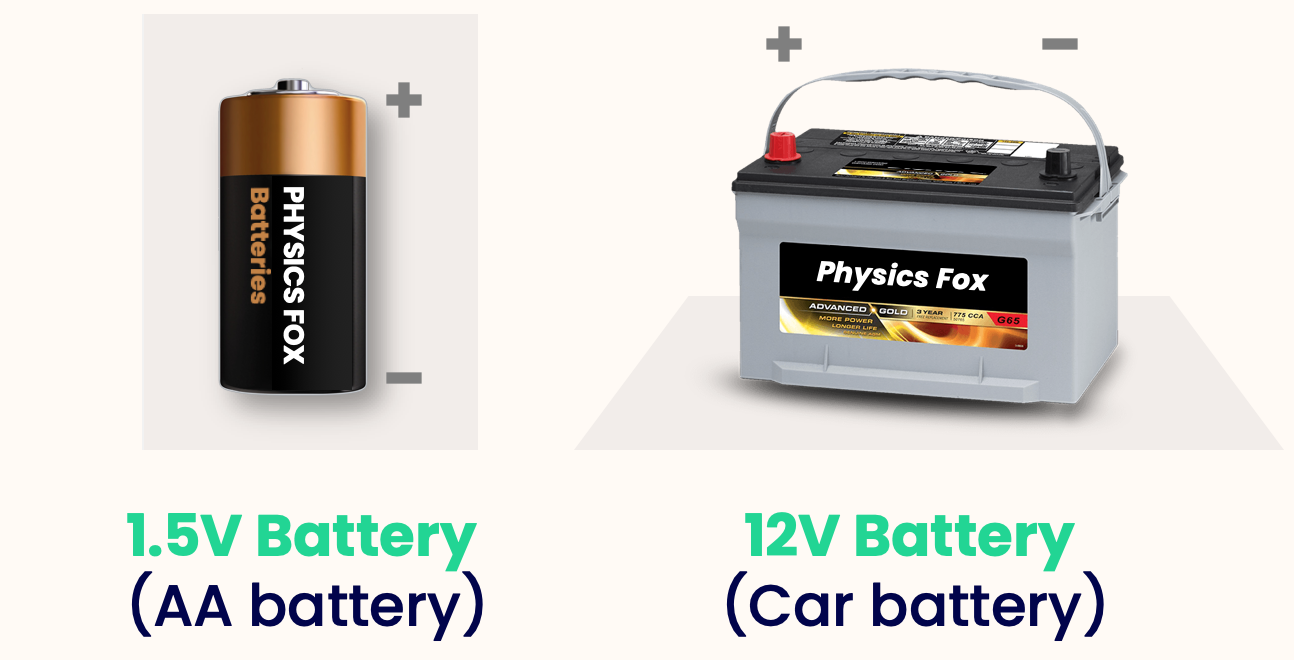She got the power in her hand
To shock you like you won't believe
Saw her in the amazon
Voltage running through her skin

In a circuit, a battery pumps electrons to a higher energy. This electrical energy has a specific name; the potential difference (also known as the voltage). Potential difference and current are the two most important concepts in electricity.

We will understand potential difference in reverse: by defining what it is, then explaining what each term means. Here's the definition: Potential difference (aka. voltage) is the difference in potential energy for each electron.

Let's think about the water circuit again.The stronger pump (right) pushes water to a higher point of the house than the weaker pump (left). In physics terms, we say the stronger pump takes water to a higher potential energy than the weaker pump. Energy is conserved, so when the water comes back down, its potential energy is used to turn the waterwheel. Therefore the more potential energy, the more energy is used to turn the waterwheel. Therefore the right waterwheel turns faster than the left one.

The water might still have some potential energy after travelling through the waterwheel. So what really matters is the difference in potential energy before and after travelling through the waterwheel.

What is true for the water circuit is also true for an electrical circuit. A stronger battery can push electrons to a higher potential energy than a weaker battery, therefore more energy can be put into electrical components (e.g. here it makes the bulb brighter).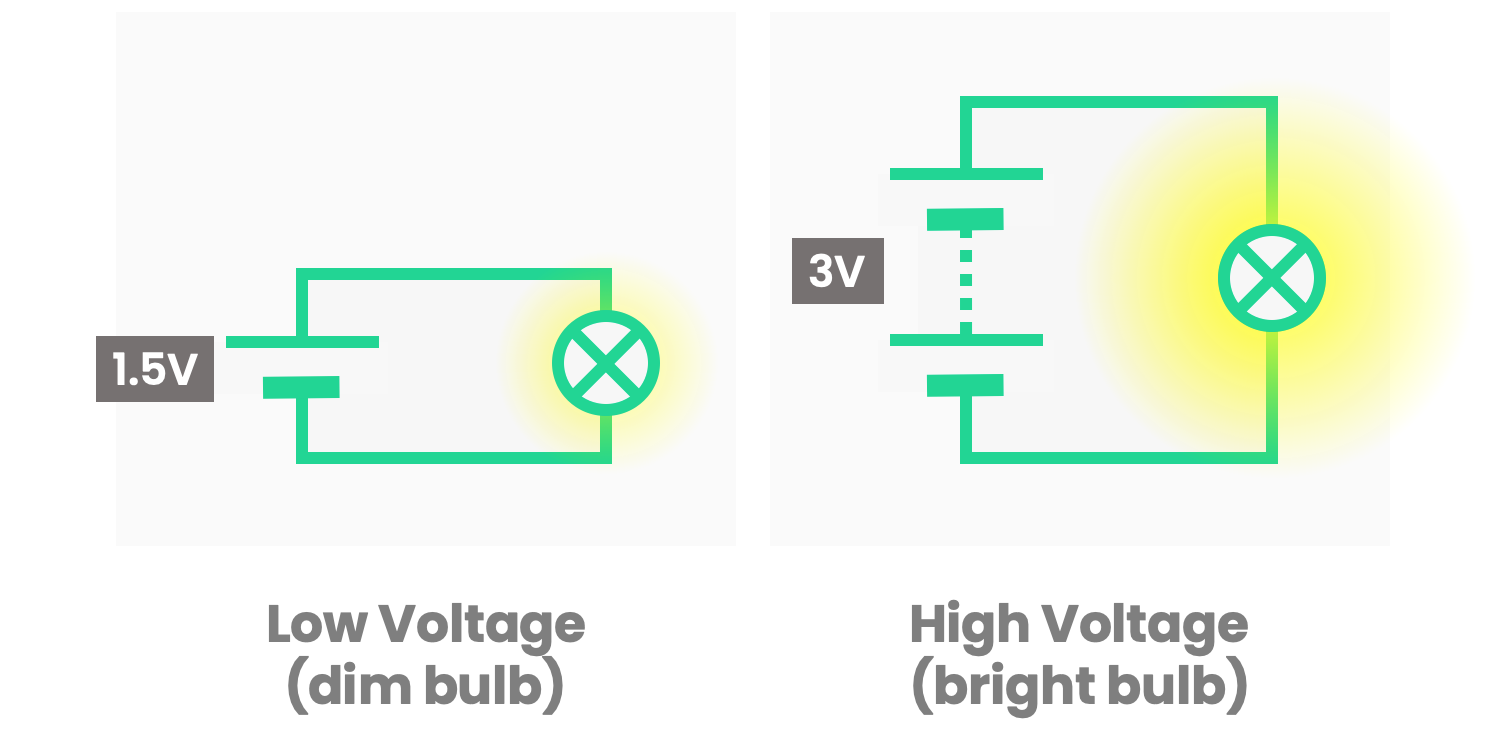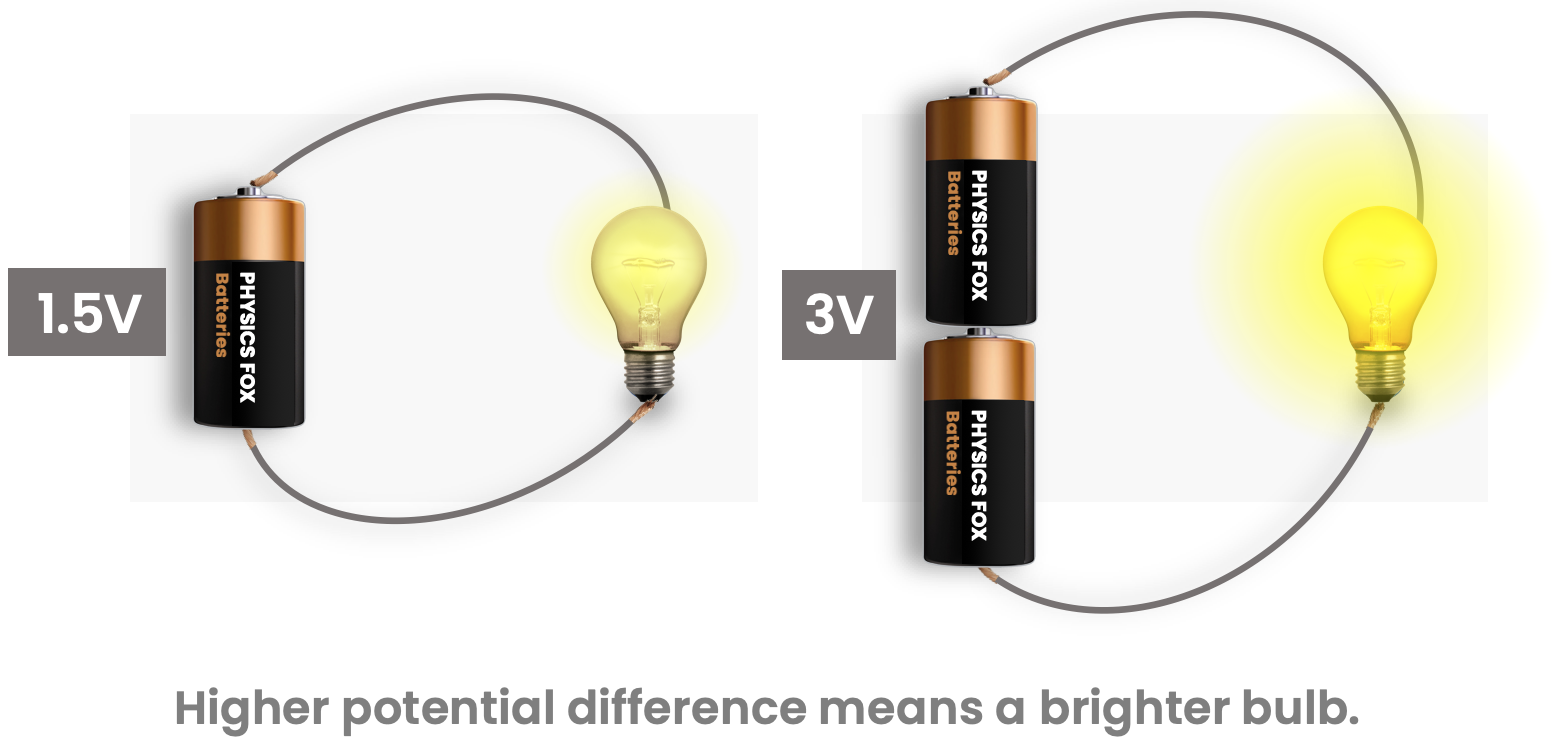A higher potential energy can also be used to power more energy-intensive devices — e.g. you can power an electric toothbrush with a 3-volt battery, but a laptop needs a 15-volt battery (more on 'volts' later).

Now we nearly understand what potential difference is — but there's still one more step.

Going back to the water circuit, we now know that to give the waterwheel more energy, we can use a stronger pump.

But there's one more way we can give the waterwheel more energy; simply having more water! (This is because the more water we have, the more weight there is pushing down on the wheel.)The pump does not care how much water is going through it — regardless of how much there is, it will always pump the water to the same height.

Similarly, a battery doesn't care how many electrons are going through it; it will always pump each electron to the same potential energy. Therefore when we define potential difference, we consider the difference in potential energy for just one electron.

Potential difference uses the symbol V, and has the SI unit of Volts (which also has the symbol V). Yes, it is confusing!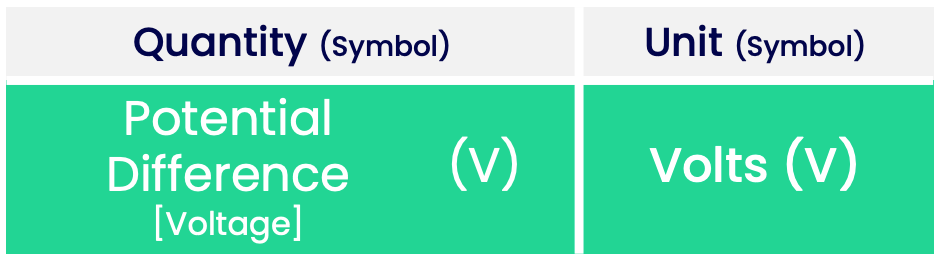For example, a car battery usually has a potential difference of 12V, and a light bulb might have a potential difference of 3V.

Voltage and volts are both named after the scientist Alessandro Giuseppe Antonio Anastasio Volta. Much like Charles-Augustin de Coulomb and André-Marie Ampère, Volta has an outrageously long name. Unlike Coulomb and Ampère, Volta does not appear on the Eiffel tower (likely because he was Italian).

I have told a slight lie in this definition of potential difference.

The real definition requires knowing what a Coulomb is — which is covered an optional section of the page about charge.

Potential difference (aka. voltage) is the difference in potential energy between two points per 1C of charge.

The idea of p.d. is the same, but this definition is more compatible with the other units we use (and is more general that just electrons — charged particles such as ions can also have a p.d.)

We also need to be specific about potential difference being between two points (this is why it's potential difference ). That's why p.d. is measured across something. e.g. The p.d. across a bulb is 3V. The bulb doesn't have a p.d. of 3V.

Quiz

### Congratulations!

5 of 5 questions completed

+ ⭐️ collected.

• You can change your display name at any time.
• at least 1 letter
• at least 1 number
• at least 8 characters